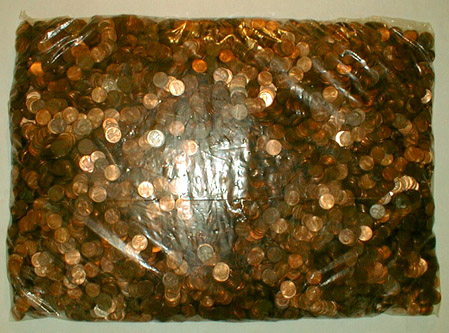to japanese | index

US\$108.56 Which Consists of 10744 Coins (Money Amount No. 31)

The U. S. coins, a plastic bag
2002

cf. 10744 and 10856 are the fourth smallest amicable pair which Euler found in 1747.
(Two numbers are called amicable if each equals to the sum of the divisors of the other.)
Divisors of 10744: 1 + 2 + 4 + 8 + 17 + 34 + 68 + 79 + 136 + 158 + 316 + 632 + 1343 + 2686 + 5372 = 10856
Divisors of 10856: 1 + 2 + 4 + 8 + 23 + 46 + 59 + 92 + 118 + 184 + 236 + 472 + 1357 + 2714 + 5428 = 10744